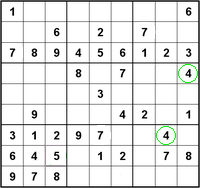Want to share your content on R-bloggers? click here if you have a blog, or here if you don't.Please note, solutions are available here.
Exercise 1
Create three vectors  `x,y,z`  with integers and each vector has 3 elements. Combine the three vectors to become a 3×3 matrix  `A`  where each column represents a vector. Change the row names to  `a,b,c`.
Think: How about each row represents a vector, can you modify your code to implement it?

Exercise 2
Please check your result from Exercise 1, using  `is.matrix(A)`. It should return  `TRUE`, if your answer is correct. Otherwise, please correct your answer. Hint: Note that  `is.matrix()`  will return  `FALSE`  on a non-matrix type of input. Eg: a vector and so on.

Exercise 3
Create a vector with 12 integers. Convert the vector to a 4*3 matrix  `B`  using  `matrix()`. Please change the column names to  `x, y, z`  and row names to  `a, b, c, d`.
The argument  `byrow`  in  `matrix()`  is set to be  `FALSE`  by default. Please change it to  `TRUE`  and print  `B`  to see the differences.

Exercise 4
Please obtain the transpose matrix of  `B`  named  `tB` .

Exercise 5
Now  `tB`  is a 3×4 matrix. By the rule of matrix multiplication in algebra, can we perform  `tB*tB`  in R language? (Is a 3×4 matrix multiplied by a 3×4 allowed?) What result would we get?

Exercise 6
As we can see from Exercise 5, we were expecting that  `tB*tB`  would not be allowed because it disobeys the algebra rules. But it actually went through the computation in R. However, as we check the output result , we notice the multiplication with a single  `*`  operator is performing the componentwise multiplication. It is not the conventional matrix multiplication. How to perform the conventional matrix multiplication in R? Can you compute matrix  `A`  multiplies  `tB` ?

Exercise 7
If we convert  `A`  to a  `data.frame`  type instead of a  `matrix` , can we still compute a conventional matrix multiplication for matrix  `A`  multiplies matrix  `A` ? Is there any way we could still perform the matrix multiplication for two  `data.frame`  type variables? (Assuming proper dimension)

Exercise 8
Extract a sub-matrix from  `B`  named  `subB` . It should be a 3×3 matrix which includes the last three rows of matrix  `B`  and their corresponding columns.

Exercise 9
Compute  `3*A` ,  `A+subB` ,  `A-subB` . Can we compute  `A+B`? Why?

Exercise 10
Generate a n * n matrix (square matrix)  `A1`  with proper number of random numbers, then generate another n * m matrix  `A2`.
If we have  `A1*M=A2`  (Here * represents the conventional multiplication), please solve for  `M`.
Hint: use the  `runif()`  and  `solve()`  functions. E.g.,  `runif(9)`  should give you 9 random numbers.

Image: “200px-Sudoku06u” by DrBorka from nl. Licensed under Wikimedia Commons.